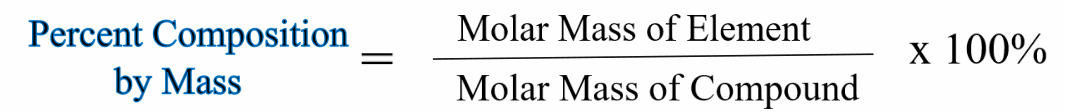# All Topics     |   Breslyn.org   |     Stoichiometry Topics

### Percent Composition by Mass

In this video you'll learn to calcuate the percent composition by mass of chemical compounds. Percent composition is a common type of problem found in chemistry courses and one you should be able to quickly solve.

### Practice Percent Comp by Mass

How to Practice Mole Conversions:
• Find the molar mass for each group of elements in the compound (e.g. all three H atoms in H3PO4).

• Find the molar mass for the entire compound.

• Divide the mass for total mass for each element by the mass of the entire compound. Multiply by 100 to get percent.

Remember, you're finding the percent composition of each element in the compound, one element at a time.

### Formula for Percent Comp by Mass

This is a general formula for finding percent composition.The molar mass of the element means the molar mass for the total number of that element in the compound. For example, in H2O2 we would find the molar mass of H and then multiply by "2" since there are two Hydrogen atoms.

More Help and Practice:

Go To Top
Subscribe